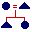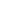Representing Anthropological Knowledge: Calculating Kinship Michael D. Fischer Analyzing and Understanding Cultural CodesKinship
Contents

 Kinship&Genealogy Kinship Introduction Learning Kinship with the Kinship Editor Use the Kinship Editor Kinship Editor Results

Kinship ContentsKinship ContentsKinship ContentsKinship Contents

Reporting Genealogical Relationships

In the previous section we defined some extended genealogical relationships that included some of the following:

mm(X,Y) :- m(Z,Y),m(X,Z).
mmm(X,Y) :- m(Z,Y),m(Q,Z),m(X,Q).
mmmm(X,Y) :- m(Z,Y),m(Q,Z),m(R,Q),m(X,R).
ffff(X,Y) :- f(Z,Y),f(Q,Z),f(R,Q),f(X,R).
fff(X,Y) :- f(Z,Y),f(Q,Z),f(X,Q).
ff(X,Y) :- f(Z,Y),f(X,Z).
mb(X,Y) :- m(Z,Y),b(X,Z).
mz(X,Y) :- m(Z,Y),z(X,Z).
fb(X,Y) :- f(Z,Y),b(X,Z).
fz(X,Y) :- f(Z,Y),z(X,Z).
mbw(X,Y) :- m(Z,Y),b(Q,Z),w(X,Q).
mzh(X,Y) :- m(Z,Y),z(Q,Z),h(X,Q).
fbw(X,Y) :- f(Z,Y),b(Q,Z),w(X,Q).
fzh(X,Y) :- f(Z,Y),z(Q,Z),h(X,Q).
fbs(X,Y) :- f(Z,Y),b(Q,Z),s(X,Q).
fbd(X,Y) :- f(Z,Y),b(Q,Z),d(X,Q).
mbs(X,Y) :- m(Z,Y),b(Q,Z),s(X,Q).
mbd(X,Y) :- m(Z,Y),b(Q,Z),d(X,Q).
zd(X,Y) :- z(Z,Y),d(X,Z).
bd(X,Y) :- b(Z,Y),d(X,Z).
zs(X,Y) :- z(Z,Y),s(X,Z).
bs(X,Y) :- b(Z,Y),s(X,Z).
ss(X,Y) :- s(Z,Y),s(X,Z).
ds(X,Y) :- d(Z,Y),s(X,Z).
sd(X,Y) :- s(Z,Y),d(X,Z).
dd(X,Y) :- d(Z,Y),d(X,Z).
scc(X,Y) :- s(Z,Y),c(Q,Z),c(X,Q).
dcc(X,Y) :- s(Z,Y),c(Q,Z),c(X,Q).
dh(X,Y) :- d(Z,Y),h(X,Z).
sw(X,Y) :- s(Z,Y),w(X,Z).
swm(X,Y) :- s(Z,Y),w(Q,Z),m(X,Q).

This is rather long-winded, since we are not capturing much of the regularity of the terms. We will keep to this schema however, to simplify the example. For a more elegant version, consult an extended example extracted from Chapter 7 of Applications in Computing for Social Anthropologists, Michael Fischer, 1994, Routledge, London.

Finding relationships between people

So far our examples will report people who have a specific relationship. Often we want to do the reverse, find all the relationships involving a single person. This is relatively straightforward if we have already defined the relationhsips we want to report.

There are many approaches. We will continue with a simple, if repetitive approach. If we define a series of rules like the following:

relate(X,Y,"f") :- f(X,Y).
relate(X,Y,"m") :- m(X,Y).
relate(X,Y,"b") :- b(X,Y).
relate(X,Y,"z") :- z(X,Y).
relate(X,Y,"s") :- s(X,Y).
relate(X,Y,"d") :- d(X,Y).
relate(X,Y,"h") :- h(X,Y).
relate(X,Y,"w") :- w(X,Y).
relate(X,Y,"ff") :- ff(X,Y).
relate(X,Y,"mm") :- mm(X,Y).
relate(X,Y,"mb") :- mb(X,Y).
...

The relate rule simply says, if the relationship on the right hand side holds, then this rule is true. This will have the effect of 'reporting' the relationship in the third position of the relate rule.

We can get a report of relationships to a person by using the query:

relate(X,mike,Rel).

Clicking the 'All Solutions' button will list any relationship mike has to anyone in the database, if that relationship has been defined by a) creating a rule for the relationship, and b) creating a corresponding relate rule.

Following is a prolog example which defines the compound relationships above, the relate rules above, and some more in the top frame. Try some of these out, by typing in a query (to the right of the "Query" button) replacing the name mike in 'relate(X,mike,Y).' to some other name, and then clicking either on the 'All Solutions' button or the 'Query' button (this gives you one result for each time you click the button). You can also enter relate(X,mike,Y), for all people mike is related to, relate(X,Y,Z). for all relationships, and relate(X,Y,"ff") for all ff relationships. Some of these will take a long time, since we have not optimised this program except for relationships to ego.

As an exercise, write a few relate clauses for mz, fz, fb, fzh, mbw, sc, dc,sw,dh. You can test your definitions by typing in a query (to the right of the "Query" button) replacing the 'mm(X,Y).' that is there.

Next section: Terminologial rules

Note for writing rules:

When writing your rules pay attention to two things:

1) make sure where you intend a variable for prolog to 'fill-in' with a name, that you use an upper-case letter for the variable name (A-Z), and

2) end your rule with a full-stop ('.'). This is VERY important.

If you get things into too big a mess, you can reload the page by clicking on the reload button of the browser while holding down the option key on a Macintosh, or the ALT key under Windows or Unix. The correct answers are incorporated into the program in next section.

Next section: Terminologial rules